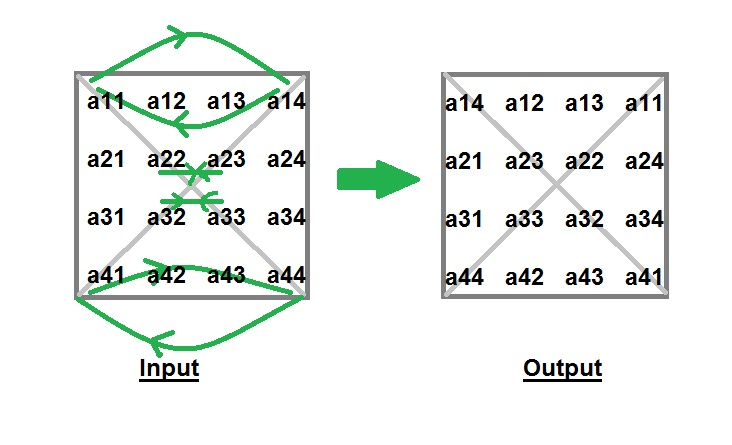# Java Program to Interchange Diagonals of Matrix

• Last Updated : 10 Jan, 2022

Given a square matrix of order n*n, you have to interchange the elements of both diagonals.
Examples :

```Input : matrix[][] = {1, 2, 3,
4, 5, 6,
7, 8, 9}
Output : matrix[][] = {3, 2, 1,
4, 5, 6,
9, 8, 7}

Input : matrix[][] = {4,  2,  3,  1,
5,  7,  6,  8,
9, 11, 10, 12,
16, 14, 15, 13}
Output : matrix[][] = {1,  2,  3,  4,
5,  6,  7,  8,
9, 10, 11, 12,
11, 14, 15, 16}```

Explanation : Idea behind interchanging diagonals of a square matrix is simple. Iterate from 0 to n-1 and for each iteration you have to swap a[i][i] and a[i][n-i-1].Time complexity : O(n)

## Java

 `// Java program to interchange ``// the diagonals of matrix``import` `java.io.*;`` ` `class` `GFG ``{``    ``public` `static` `int` `N = ``3``;``     ` `    ``// Function to interchange diagonals``    ``static` `void` `interchangeDiagonals(``int` `array[][])``    ``{``        ``// swap elements of diagonal``        ``for` `(``int` `i = ``0``; i < N; ++i)``            ``if` `(i != N / ``2``)``            ``{``                ``int` `temp = array[i][i];``                ``array[i][i] = array[i][N - i - ``1``];``                ``array[i][N - i - ``1``] = temp;``            ``}`` ` `        ``for` `(``int` `i = ``0``; i < N; ++i)``        ``{``            ``for` `(``int` `j = ``0``; j < N; ++j)``                ``System.out.print(array[i][j]+``" "``);``            ``System.out.println();``        ``}``    ``}``     ` `    ``// Driver Code``    ``public` `static` `void` `main (String[] args) ``    ``{``        ``int` `array[][] = { {``4``, ``5``, ``6``},``                        ``{``1``, ``2``, ``3``},``                        ``{``7``, ``8``, ``9``}``                        ``};``        ``interchangeDiagonals(array);``    ``}``}`` ` `// This code is contributed by Pramod Kumar`

Output:

``` 6 5 4
1 2 3
9 8 7```

Please refer complete article on Program to Interchange Diagonals of Matrix for more details!

My Personal Notes arrow_drop_up